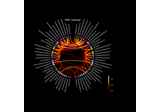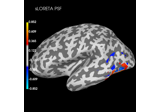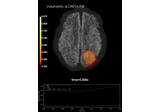mne.minimum_norm.get_point_spread(resmat, src, idx, mode=None, *, n_comp=1, norm=False, return_pca_vars=False, vector=False, verbose=None)[source]#

Get point-spread (PSFs) functions for vertices.

Parameters
resmat`array`, shape (n_dipoles, n_dipoles)

Forward Operator.

srcinstance of `SourceSpaces` | instance of `InverseOperator` | instance of `Forward`

Source space used to compute resolution matrix. Must be an InverseOperator if `vector=True` and a surface source space is used.

idx

Source for indices for which to compute PSFs or CTFs. If mode is None, PSFs/CTFs will be returned for all indices. If mode is not None, the corresponding summary measure will be computed across all PSFs/CTFs available from idx. Can be:

• list of integers : Compute PSFs/CTFs for all indices to source space vertices specified in idx.

• list of Label : Compute PSFs/CTFs for source space vertices in specified labels.

mode`None` | ‘mean’ | ‘max’ | ‘svd’

Compute summary of PSFs/CTFs across all indices specified in ‘idx’. Can be:

• None : Output individual PSFs/CTFs for each specific vertex (Default).

• ‘mean’ : Mean of PSFs/CTFs across vertices.

• ‘max’ : PSFs/CTFs with maximum norm across vertices. Returns the n_comp largest PSFs/CTFs.

• ‘svd’ : SVD components across PSFs/CTFs across vertices. Returns the n_comp first SVD components.

n_comp`int`

Number of PSF/CTF components to return for mode=’max’ or mode=’svd’. Default n_comp=1.

norm`None` | ‘max’ | ‘norm’

Whether and how to normalise the PSFs and CTFs. This will be applied before computing summaries as specified in ‘mode’. Can be:

• None : Use un-normalized PSFs/CTFs (Default).

• ‘max’ : Normalize to maximum absolute value across all PSFs/CTFs.

• ‘norm’ : Normalize to maximum norm across all PSFs/CTFs.

return_pca_vars`bool`

Whether or not to return the explained variances across the specified vertices for individual SVD components. This is only valid if mode=’svd’. Default return_pca_vars=False.

vector`bool`

Whether to return PSF/CTF as vector source estimate (3 values per location) or source estimate object (1 intensity value per location). Only allowed to be True if corresponding dimension of resolution matrix is 3 * n_dipoles. Defaults to False.

New in v1.2.

verbose

Control verbosity of the logging output. If `None`, use the default verbosity level. See the logging documentation and `mne.verbose()` for details. Should only be passed as a keyword argument.

Returns
stcsinstance of `SourceEstimate` | `list` of instances of `SourceEstimate`

The PSFs or CTFs as STC objects. All PSFs/CTFs will be returned as successive samples in STC objects, in the order they are specified in idx. STCs for different labels willbe returned as a list. If resmat was computed with n_orient_inv==3 for CTFs or n_orient_fwd==3 for PSFs then 3 functions per vertex will be returned as successive samples (i.e. one function per orientation). If vector=False (default) and resmat was computed with n_orient_inv==3 for PSFs or n_orient_fwd==3 for CTFs, then the three values per vertex will be combined into one intensity value per vertex in a SourceEstimate object. If vector=True, PSFs or CTFs with 3 values per vertex (one per orientation) will be returned in a VectorSourceEstimate object.

pca_vars`array`, shape (n_comp,) | `list` of `array`

The explained variances of the first n_comp SVD components across the PSFs/CTFs for the specified vertices. Arrays for multiple labels are returned as list. Only returned if mode=’svd’ and return_pca_vars=True.

## Examples using `mne.minimum_norm.get_point_spread`#Visualize source leakage among labels using a circular graph

Visualize source leakage among labels using a circular graphPlot point-spread functions (PSFs) and cross-talk functions (CTFs)

Plot point-spread functions (PSFs) and cross-talk functions (CTFs)Plot point-spread functions (PSFs) for a volume

Plot point-spread functions (PSFs) for a volume